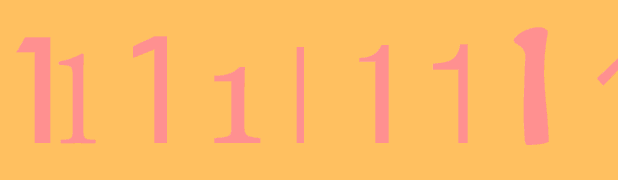# Divisible By 41Consider all values of $n$ such that the number $111\ldots 111$ in which the number 1 occurs $n$ times is divisible by 41.

What is the largest integer which must be a factor of $n$?

×